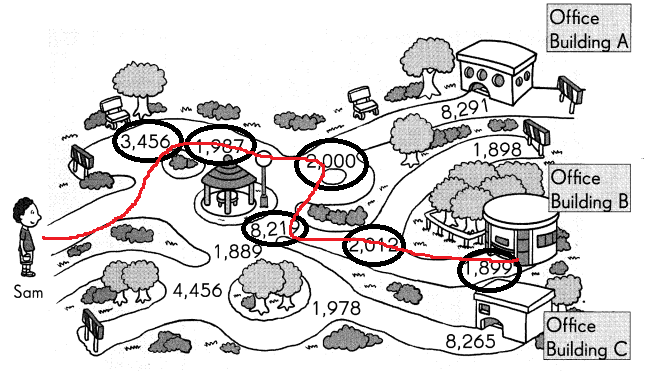# Math in Focus Grade 3 Chapter 1 Practice 3 Answer Key Comparing and Ordering Numbers

Go through the Math in Focus Grade 3 Workbook Answer Key Chapter 1 Practice 3 Comparing and Ordering Numbers to finish your assignments.

## Math in Focus Grade 3 Chapter 1 Practice 3 Answer Key Comparing and Ordering Numbers

Compare. Fill in each blank.

Question 1.
Which number is greater?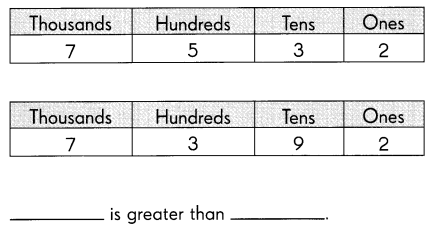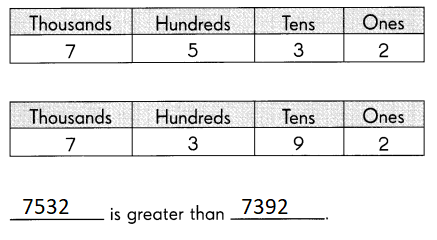Explanation:
First we have to compare the thousands
if thousands are equal we have to
compare the hundreds

Question 2.
Which number is less?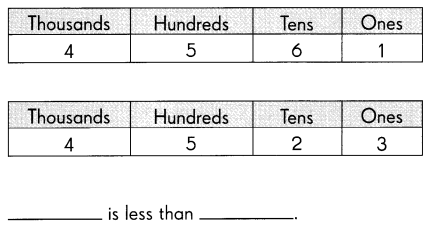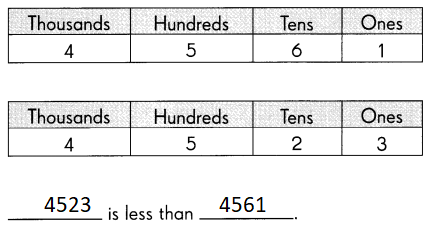Explanation:
First we have to compare the thousands
if thousands are equal we have to
compare the hundreds
if hundreds are same we have to compare the tens

Compare. Write > or <.

Question 3.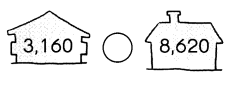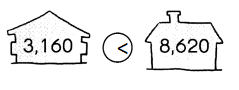Explanation:
First we have to compare the thousands
if thousands are equal we have to
compare the hundreds
if hundreds are same we have to compare the tens

Question 4.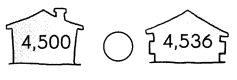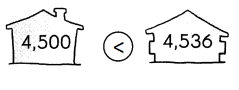Explanation:
First we have to compare the thousands
if thousands are equal we have to
compare the hundreds
if hundreds are same we have to compare the tens

Question 5.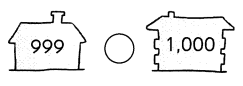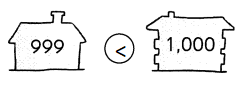Explanation:
First we have to compare the thousands
if thousands are equal we have to
compare the hundreds
if hundreds are same we have to compare the tens

Question 6.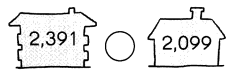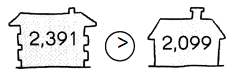Explanation:
First we have to compare the thousands
if thousands are equal we have to
compare the hundreds
if hundreds are same we have to compare the tens

Circle the greater number.

Question 7.
8,687 8,987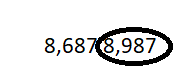Explanation:
First we have to compare the thousands
if thousands are equal we have to
compare the hundreds
if hundreds are same we have to compare the tens

Question 8.
1,251 1,231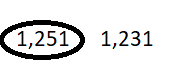Explanation:
First we have to compare the thousands
if thousands are equal we have to
compare the hundreds
if hundreds are same we have to compare the tens

Circle the number that is less.

Question 9.
2,012 200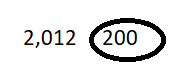Explanation:
First we have to compare the thousands
if thousands are equal we have to
compare the hundreds
if hundreds are same we have to compare the tens

Question 10.
7,400 7,402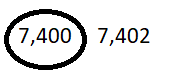Explanation:
First we have to compare the thousands
if thousands are equal we have to
compare the hundreds
if hundreds are same we have to compare the tens

Circle the greatest number and underline the least number.

Question 11.
6,963 6,639 6,696 6,993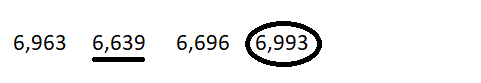Explanation:
First we have to compare the thousands
if thousands are equal we have to
compare the hundreds
if hundreds are same we have to compare the tens

Order each set of numbers from least to greatest.

Question 12.
2,340 989 4,001
989 2,340 4,001
Explanation:
ordered from least to greatest
First we have to compare the thousands
if thousands are equal we have to
compare the hundreds
if hundreds are same we have to compare the tens

Question 13.
2,456 1,456 6,456
1,456 2,456 6,456
Explanation:
ordered from least to greatest
First we have to compare the thousands
if thousands are equal we have to
compare the hundreds
if hundreds are same we have to compare the tens

Question 14.
6,359 6,059 6,759
6,059 6,359 6,759
Explanation:
ordered from least to greatest
First we have to compare the thousands
if thousands are equal we have to
compare the hundreds
if hundreds are same we have to compare the tens

Question 15.
3,052 3,057 3,050
3,050 3,052 3,057
Explanation:
ordered from least to greatest
First we have to compare the thousands
if thousands are equal we have to
compare the hundreds
if hundreds are same we have to compare the tens

Order each set of numbers from greatest to least.

Question 16.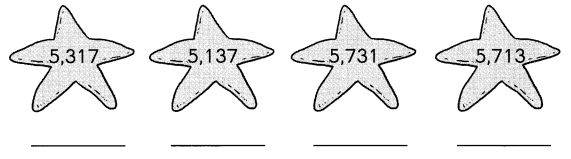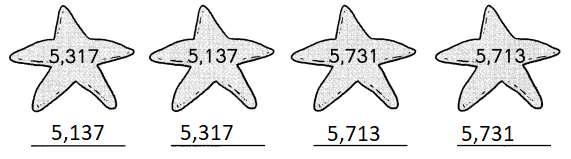Explanation:
Ordered from greatest to least
First we have to compare the thousands
if thousands are equal we have to
compare the hundreds
if hundreds are same we have to compare the tens

Question 17.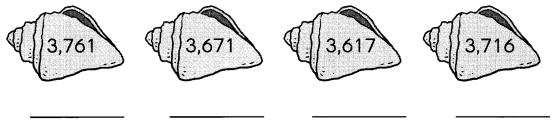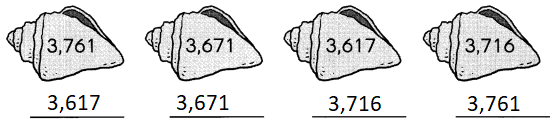Explanation:
Ordered from greatest to least
First we have to compare the thousands
if thousands are equal we have to
compare the hundreds
if hundreds are same we have to compare the tens

Question 18.
1 more than 6,348 is ____.
6348 + 1 = 6349
Explanation:
To find 1 more we have to add that number with 1

Question 19.
1,000 more than 3217 is ___.
3217 + 1000 = 4217
Explanation:
To find 1000 more we have to add that number with 1000

Question 20.
100 less than 5,608 is ___.
5608 – 100 = 5508
Explanation:
To find 100 less we have to subtract that number with 100

Question 21.
10 less than 2,000 is ___.
2000 – 10 = 1990
Explanation:
To find 10 less we have to subtract that number with 10

Look for a pattern. Fill in the missing numbers.

Question 22.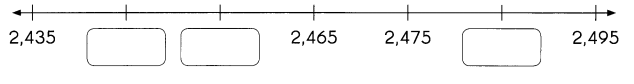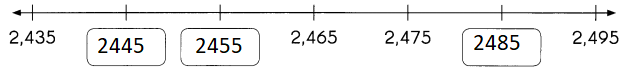Explanation:
The thousand, hundreds and ones place are remaining same
tens place is increasing by 10

Question 23.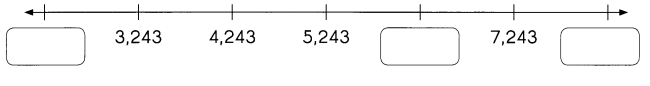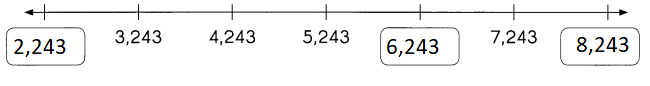Explanation:
The thousands place change by 1000 for every pattern
hundreds, tens and ones place remains same.

Question 24.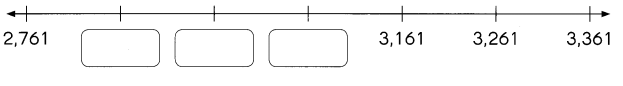Explanation:
The hundreds place increase by 100 for every pattern
thousands, tens and ones place remains same.

Look for a pattern. Fill in the missing numbers.

Question 25.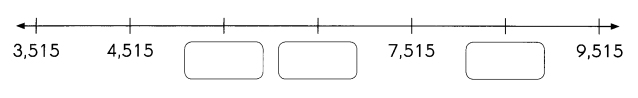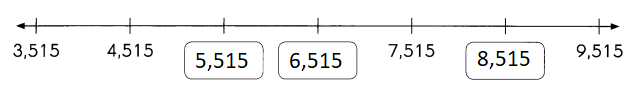Explanation:
In the above pattern it increase by 1000
hundreds, tens and ones place remains same.

Question 26.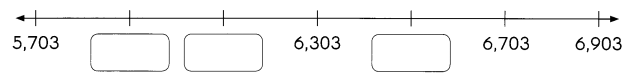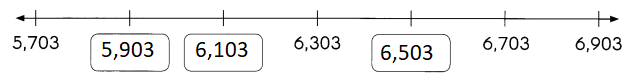Explanation:
In the above pattern the hundred place increase by 200
thousands, tens and ones place remains same.

Question 27.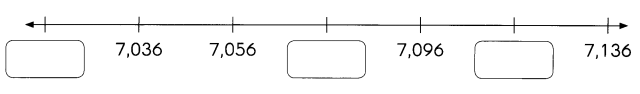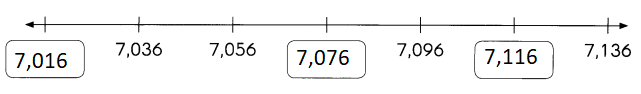Explanation:
In the above pattern the tens place increase by 20 for every number
thousands, hundreds and ones place remains same.

Complete each number pattern.

Question 28.
________ ________ ________ 5,755 5,765 5,775
5,725  5,735  5,745  5,755  5,765  5,775
Explanation:
In the above pattern the tens place increase by 10 for every number

Question 29.
8,625 8,725 ____ ____ 9,025 9,125
8,625 8,725 8,825 8,925 9,025 9,125
Explanation:
In the above pattern the hundreds place increase by 100 for every number

Question 30.
862 962 ____ 1,162 1,262
862 962 1,062 1,162 1,262
Explanation:
In the above pattern the hundreds place increase by 100 for every number

Question 31.
6,315 6,215 6,115 ___ ____ ____
6,315 6,215 6,115 6,015  5,915 5,815
Explanation:
In the above pattern the hundred place decrease by 100 for every number

Help Sam follow the correct numbers on the map to get to his mother’s office. Write your answers in the boxes.

Question 32.
Which is the least: 3,456 8,265 or 4,456? ____
3,456
Explanation:
3,456 is the least number in the pattern

Question 33.
Which is the greatest: 1,978 1,987 or 1 ,889? _______
1,987
Explanation:
1,987 is the greatest number in the pattern

Question 34.
Complete the number pattern. _______
1,980 1,990 ___ 2,010
1,980 1,990 2,000 2,010
Explanation:
The tens place change by 10 number for every pattern.

Question 35.
Which is less: 8,219 or 8,291? _____
8,219
Explanation:
8,219 is less in the pattern.

Question 36.
100 more than 1,912 is __________
2,012
Explanation:
100 more than 1,912 is 2,012

Question 37.
What number is missing? _____
1,901 1,900 ___ 1,898
1,901 1,900 1,899 1,898
Explanation:
In The above pattern the number decrease by one in ones place.

Using the numbers in the boxes, color the route that Sam takes to his mother’s office.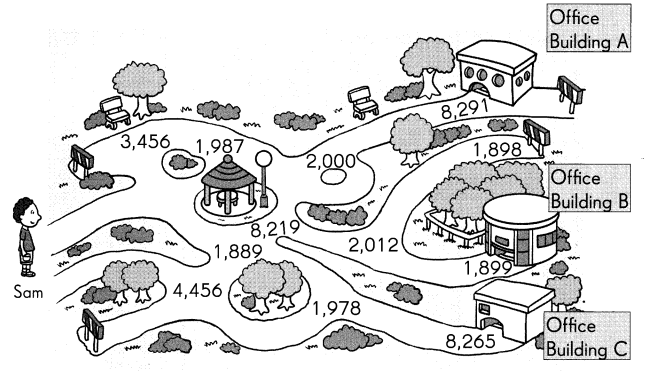Question 38.
Which office buildings does he go to? Office building _____